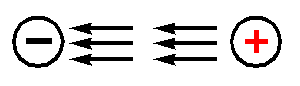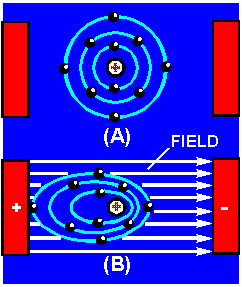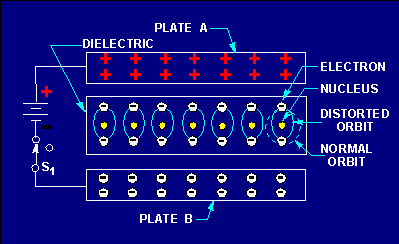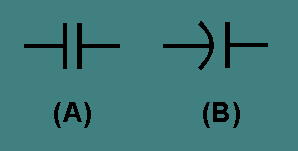CAPACITANCE - Page 3Custom SearchCAPACITANCE LEARNING OBJECTIVES Upon completion of this chapter you will be able to: Define the terms "capacitor" and "capacitance." State four characteristics of electrostatic lines of force. State the effect that an electrostatic field has on a charged particle. State the basic parts of a capacitor. Define the term "farad". State the mathematical relationship between a farad, a microfarad, and a picofarad. State three factors that affect the value of capacitance. Given the dielectric constant and the area of and the distance between the plates of a capacitor, solve for capacitance. State two types of power losses associated with capacitors. Define the term "working voltage" of a capacitor, and compute the working voltage of a capacitor. State what happens to the electrons in a capacitor when the capacitor is charging and when it is discharging. State the relationship between voltage and time in an RC circuit when the circuit is charging and discharging. State the relationship between the voltage drop across a resistor and the source voltage in an RC circuit. Given the component values of an RC circuit, compute the RC time constant. Use the universal time constant chart to determine the value of an unknown capacitor in an RC circuit. Calculate the value of total capacitance in a circuit containing capacitors of known value in series. Calculate the value of total capacitance in a circuit containing capacitors of known value in parallel. State the difference between different types of capacitors. Determine the electrical values of capacitors using the color code. In the previous chapter you learned that inductance is the property of a coil that causes electrical energy to be stored in a magnetic field about the coil. The energy is stored in such a way as to oppose any change in current. CAPACITANCE is similar to inductance because it also causes a storage of energy. A CAPACITOR is a device that stores electrical energy in an ELECTROSTATIC FIELD. The energy is stored in such a way as to oppose any change in voltage. Just how capacitance opposes a change in voltage is explained later in this chapter. However, it is first necessary to explain the principles of an electrostatic field as it is applied to capacitance. Q.1 Define the terms "capacitor" and "capacitance." THE ELECTROSTATIC FIELD You previously learned that opposite electrical charges attract each other while like electrical charges repel each other. The reason for this is the existence of an electrostatic field. Any charged particle is surrounded by invisible lines of force, called electrostatic lines of force. These lines of force have some interesting characteristics: They are polarized from positive to negative. They radiate from a charged particle in straight lines and do not form closed loops. They have the ability to pass through any known material. They have the ability to distort the orbits of tightly bound electrons. Examine figure 3-1. This figure represents two unlike charges surrounded by their electrostatic field. Because an electrostatic field is polarized positive to negative, arrows are shown radiating away from the positive charge and toward the negative charge. Stated another way, the field from the positive charge is pushing, while the field from the negative charge is pulling. The effect of the field is to push and pull the unlike charges together. Figure 3-1. - Electrostatic field attracts two unlike charged particles.In figure 3-2, two like charges are shown with their surrounding electrostatic field. The effect of the electrostatic field is to push the charges apart. Figure 3-2. - Electrostatic field repels two like charged particles.If two unlike charges are placed on opposite sides of an atom whose outermost electrons cannot escape their orbits, the orbits of the electrons are distorted as shown in figure 3-3. Figure 3-3(A) shows the normal orbit. Part (B) of the figure shows the same orbit in the presence of charged particles. Since the electron is a negative charge, the positive charge attracts the electrons, pulling the electrons closer to the positive charge. The negative charge repels the electrons, pushing them further from the negative charge. It is this ability of an electrostatic field to attract and to repel charges that allows the capacitor to store energy. Figure 3-3. - Distortion of electron orbital paths due to electrostatic force.Q.2 State four characteristics of electrostatic lines of force. Q.3 An electron moves into the electrostatic field between a positive charge and a negative charge. Toward which charge will the electron move? THE SIMPLE CAPACITOR A simple capacitor consists of two metal plates separated by an insulating material called a dielectric, as illustrated in figure 3-4. Note that one plate is connected to the positive terminal of a battery; the other plate is connected through a closed switch (S1) to the negative terminal of the battery. Remember, an insulator is a material whose electrons cannot easily escape their orbits. Due to the battery voltage, plate A is charged positively and plate B is charged negatively. (How this happens is explained later in this chapter.) Thus an electrostatic field is set up between the positive and negative plates. The electrons on the negative plate (plate B) are attracted to the positive charges on the positive plate (plate A). Figure 3-4. - Distortion of electron orbits in a dielectric.Notice that the orbits of the electrons in the dielectric material are distorted by the electrostatic field. The distortion occurs because the electrons in the dielectric are attracted to the top plate while being repelled from the bottom plate. When switch S1 is opened, the battery is removed from the circuit and the charge is retained by the capacitor. This occurs because the dielectric material is an insulator, and the electrons in the bottom plate (negative charge) have no path to reach the top plate (positive charge). The distorted orbits of the atoms of the dielectric plus the electrostatic force of attraction between the two plates hold the positive and negative charges in their original position. Thus, the energy which came from the battery is now stored in the electrostatic field of the capacitor. Two slightly different symbols for representing a capacitor are shown in figure 3-5.Notice that each symbol is composed of two plates separated by a space that represents the dielectric. The curved plate in (B) of the figure indicates the plate should be connected to a negative polarity. Figure 3-5. - Circuit symbols for capacitors.Q.4 What are the basic parts of a capacitor?Integrated Publishing, Inc. - A (SDVOSB) Service Disabled Veteran Owned Small Business